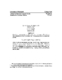## On the Powers of a Matrix with Perturbations##### Files
CS-TR-4317.ps(151.94 KB)
Let $A$ be a matrix of order $n$. The properties of the powers $A^{k}$ of $A$ have been extensively studied in the literature. This paper concerns the perturbed powers $P_{k} = (A+E_{k})(A+E_{k-1})\cdots(A+E_{1}),$ where the $E_{k}$ are perturbation matrices. We will treat three problems concerning the asymptotic behavior of the perturbed powers. First, determine conditions under which $P_{k}\rightarrow 0$. Second, determine the limiting structure of $P_{k}$. Third, investigate the convergence of the power method with error: that is, given $u_{1}$, determine the behavior of $u_{k} = \nu_{k}P_{k}u_{1}$, where $\nu_{k}$ is a suitable scaling factor. (Also UMIACS-TR-2001-91)